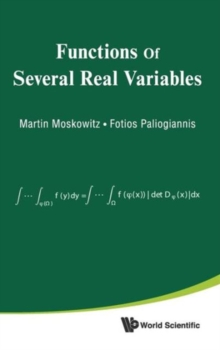Supporting your high street Find out how »
• My AccountFunctions Of Several Real Variables Hardback

Description

This book begins with the basics of the geometry and topology of Euclidean space and continues with the main topics in the theory of functions of several real variables including limits, continuity, differentiation and integration.

All topics and in particular, differentiation and integration, are treated in depth and with mathematical rigor.

The classical theorems of differentiation and integration such as the Inverse and Implicit Function theorems, Lagrange's multiplier rule, Fubini's theorem, the change of variables formula, Green's, Stokes' and Gauss' theorems are proved in detail and many of them with novel proofs.

The authors develop the theory in a logical sequence building one result upon the other, enriching the development with numerous explanatory remarks and historical footnotes.

A number of well chosen illustrative examples and counter-examples clarify matters and teach the reader how to apply these results and solve problems in mathematics, the other sciences and economics.Each of the chapters concludes with groups of exercises and problems, many of them with detailed solutions while others with hints or final answers.

More advanced topics, such as Morse's lemma, Sard's theorem , the Weierstrass approximation theorem, the Fourier transform, Vector fields on spheres, Brouwer's fixed point theorem, Whitney's embedding theorem, Picard's theorem, and Hermite polynomials are discussed in stared sections.

Information

• Format: Hardback
• Pages: 732 pages
• Publisher: World Scientific Publishing Co Pte Ltd
• Publication Date:
• Category: Calculus & mathematical analysis
• ISBN: 9789814299268

Other Formats

£123.00

£106.39

on all orders

Pick up orders

from local bookshops

£61.00

£50.59